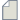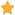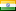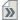###Author Topic: Hydrogen Molecule Dissociation energy calculation  (Read 38 times)

0 Members and 1 Guest are viewing this topic.

####AsifShah

• Regular ATK user
•• Posts: 23
• Country:• Reputation: 0##### Hydrogen Molecule Dissociation energy calculation
« on: June 14, 2022, 14:56 »
I am calculating the dissociation energy of Hydrogen molecule.
The formula used is E(disso)=E(H2)-2E(H)
where E(H2) is the total energy calculated using two methods as:
1. Spin unpolarised/polarized using B3LYP functionals giving energies - 32, -32 respectively.
2. Spin unpolarised/polarised using PBE functionals giving energies - 31.651, -27.181 respectively.
Since H2 is spin unpolarised molecule & when choosing unpolarised/polarised using B3LYP both give same energy -32 which makes sense but why does PBE give different results for spin polarised & spin unpolarised?
« Last Edit: June 14, 2022, 15:00 by AsifShah »